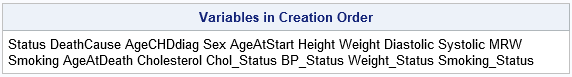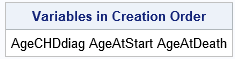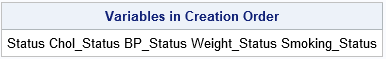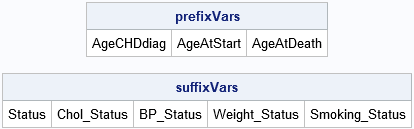A common task in SAS programming is to specify a list of variables that satisfy some pattern. You can specify lists for the KEEP= or DROP= data set options, and you can use lists of variables on many SAS statements such as the VAR and MODEL statements. Although SAS has built-in support for some patterns (like variables that start with the same prefix), you might want to match variable names to less-common patterns. In those situations, you can use regular expressions to match variable names by using the PRXMATCH function in Base SAS. The PRXMATCH function is one of several functions in SAS that support Perl regular expressions (PRX).

### Built-in support for specifying variables in SAS

In a previous article, I discussed six different ways to create a list of variable names in SAS. Of these, the most common are

• The colon operator for specifying names that have a common prefix. For example, AGE: specifies variables that begin with the prefix "age" (recall that SAS variable names are case insensitive).
• The dash operator for specifying a sequence of variable names that have the same prefix and a numerical suffix. For example, X1-X5 matches the variables X1, X2, X3, X4, and X5.

For example, the following table shows variables in the Sashelp.Heart data set:

`proc contents data=Sashelp.Heart short varnum; run;`You can see that several variables begin with the prefix 'Age'. By using the colon operator (Age:) you can match all variables that match the prefix, such as in the following example:

```title "Colon (Prefix) Variable Names"; data PrefixVars; set Sashelp.Heart(keep=Age:); run; proc contents short varnum; run;```### Specifying variables that have a common suffix

Several of the variables in the Sashelp.Heart data end with the suffix 'Status'. Unfortunately, there is no built-in operator in SAS to match a suffix such as 'Status'. However, Bruno Mueller and Mark Jordan have provided a SAS macro that uses regular expressions to select variables that match any pattern. The list of variables is returned as a text string, so you can use it in the usual places, such as the KEEP= options or a VAR statement. The following example assumes you have downloaded and run the definition of the %varListPattern macro.

```title "Suffix Variable Names"; data SuffixVars; set Sashelp.Heart(keep=%varListPattern(sashelp.Heart,*status)); run; proc contents short varnum; run;```This macro fills a need, and I like it a lot. In addition to matching variables that share a common suffix, you can also use the macro to find variables that match other patterns. For example, you can use the pattern "*at*" to match variables that have the string "at" anywhere in their name. In addition to the "status" variables found above, that pattern also matches the variables DeathCause, AgeAtStart, and AgeAtDeath.

Some programmers don't realize that all SAS procedures support data set options (such as KEEP= and DROP=) when you specify the name of a data set in a procedure. That means that you can use the built-in pattern matching and the %varListPattern macro throughout SAS. For example, here is how you would read a set of prefix-variables and a set of suffix-variables into SAS/IML matrices:

```proc iml; use Sashelp.Heart(keep=Age:); /* use the coon operator to match prefixes */ read all var _ALL_ into X[colname=prefixVars]; close; print prefixVars;   use Sashelp.Heart(keep=%varListPattern(sashelp.Heart,*status)); /* use macro to match suffixes */ read all var _ALL_ into C[colname=suffixVars]; close; print suffixVars;```### Extract a vector of strings that match a pattern

At its heart, the %varListPattern macro calls the PRXMATCH function. You can use the PRXMATCH function to determine whether a variable name (in fact, any string!) matches a pattern that is specified by a regular expression. You can use the PRXMATCH function when the names of variables are in a data set. You can then use PROC SQL to create a macro variable that contains a space-separated list of all variables that match the pattern.

I needed this functionality recently when I was writing a SAS/IML function and needed to select all strings that had a common suffix. To understand the next example, recall the following SAS/IML programming features:

The following SAS/IML functions construct patterns like /^PREFIX/i and /.*SUFFIX\$/i and use the PRXMATCH function to find strings that match the patterns. To demonstrate the function, the program uses the variable names in the Sashelp.Heart data.

```proc iml; /* Use PRXMATCH to find strings with a common prefix */ start MatchPrefix(prefix, str); re = '/^' + strip(prefix) + '/i'; /* beginning of word, case insensitive */ idx = loc(prxmatch(re, str)); if ncol(idx)=0 then return( {} ); /* return empty matrix */ return( str[idx] ); finish;   /* Use PRXMATCH to find strings with a common suffix */ start MatchSuffix(suffix, str); re = '/.*' + strip(suffix) + '\$/i'; /* end of word, case insensitive */ idx = loc(prxmatch(re, right(str))); /* shift right so no blank spaces at end */ if ncol(idx)=0 then return( {} ); /* return empty matrix */ return( str[idx] ); finish;   Variable = contents( "Sashelp", "Heart" ); /* get variable names in data order */ prefixVars = MatchPrefix("Age", Variable); suffixVars = MatchSuffix("status", Variable); print prefixVars, suffixVars;```

I emphasize that although this example uses variable names as the strings, you can use the PRXMATCH function to search for patterns in arbitrary strings. In a similar way, you can construct other functions that find strings that match any regular expression that you can construct.

In summary, regular expressions in SAS are a powerful feature for matching strings that contain some pattern of characters. The examples in this article use simple regular expressions to find all variables that contain a common prefix (same as the built-in colon operator) or a common suffix. For many SAS procedures that require a list of variable names, you can use the %varListPattern macro to generate the variable list. For other applications, you can call the PRXMATCH function directly.

For an introduction to regular expressions in SAS, see "An Introduction to Perl Regular Expressions in SAS 9" (Cody, 2004) and "The Basics of the PRX Functions" (Cassell, 2007).

ShareDistinguished Researcher in Computational Statistics

Rick Wicklin, PhD, is a distinguished researcher in computational statistics at SAS and is a principal developer of SAS/IML software. His areas of expertise include computational statistics, simulation, statistical graphics, and modern methods in statistical data analysis. Rick is author of the books Statistical Programming with SAS/IML Software and Simulating Data with SAS.

1.Rick,
No need '.*' , just 'age\$' is enough.
But need watch the trail blanks of variable ,
Here I used STRIP() ,while you used RIGHT() .

data _null_;
input name \$20.;
if prxmatch('/age\$/i',strip(name)) then flag='Y';
else flag='N';
put (_all_) (:) ;
cards;
Tom_age
Arthur_Age
King_AAA
;

•Thanks for writing. I put the leading '.*' in because I often use concatenation to create the string dynamically. For example, here is how you can find the variables that match any of three different suffixes by using a loop:

```  data Suffix; array suf \$ ('tolic', 'status', 'ing'); /* match any of these sufixes */ set Have(keep=Variable); keep = 0; do i = 1 to dim(suf); re = catt('/.*', suf[i], '\$/i'); /* '/.*ABC\$/i' */ keep = bor(keep, prxmatch(re, right(Variable)) ); end; if keep; run; proc print; var Variable; run;```